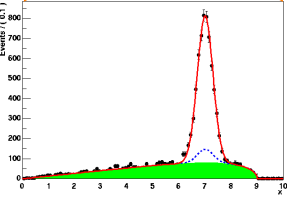# RooArgusBG reflection

Dear Experts,

I have been trying to modeling a signal + background distribution using the RooArgusBG PDF which is ideal for the fit, taken the plot of reference, how can I reflect the Argus distribution to the opposite side, I mean so that the fall that is seen on the right is on the left.Many thanks

Hi @jbarajas, welcome to the ROOT forum!

That’s a very good question, and there is a good solution. In general, in RooFit you can use the RooFormulaVar for variable transformations. Then, you define the pdf on the transformed variable. However, you’ll have to pay a hefty performance penalty in fitting, because RooFit does not know anymore how to do the pdf normalization without numeric integrals in this case.

That’s why I recommend you to use the RooLinearVar in this case. Here is an example:

``````// Observable:
RooRealVar x("x", "x", 0, 10);

// Using a variable transformation with the RooFormulaVar would do the trick.
// However, RooFit doesn't know how to analytically do the normalization over a
// variable that is transformed generally like this. It is commented out
// because there is a better solution. You can try it out, and you will see
// messages about numeric integration in the terminal output.
//
//   RooFormulaVar xMirrored("x_mirrored", "10 - x", x);

// This is the key: define the pdfs on a linearly shifted version of the
// observable. Only in this case, RooFit still knows how to normalize the pdf
// without numeric integrals.
RooLinearVar xMirrored("x_mirrored", "x_mirrored", x, RooConst(-1.0), RooConst(10.0));

// Parameters:
RooRealVar sigmean("sigmean", "sigmean", 3.0, 0.0, 10.0);
RooRealVar sigwidth("sigwidth", "sigwidth", 0.3, 0.1, 10.0);

// Build a Gaussian PDF. Note that here we don't use the shifted variable:
RooGaussian signalModel("signal", "signal", x, sigmean, sigwidth);

// Build Argus background PDF:
RooRealVar argpar("argpar", "argpar", -0.5, -10., -0.01);
RooArgusBG background("background", "background", xMirrored, RooConst(9.0), argpar);

// Construct a signal and background PDF:
RooRealVar nsig("nsig", "nsig", 400, 0., 10000);
RooRealVar nbkg("nbkg", "nkbkg", 800, 0., 10000);
RooAddPdf model("model", "model", {signalModel, background}, {nsig, nbkg});

// Generate a toy MC sample from composite PDF:
std::unique_ptr<RooDataSet> data{model.generate(x, 2000)};

// Perform extended ML fit of composite PDF to toy data:
std::unique_ptr<RooFitResult> res{model.fitTo(*data, PrintLevel(-1), Minimizer("Minuit"), Save())};
res->Print();

// Create a RooPlot to draw on. We don't manage the memory of the returned
// pointer. Instead we let it leak such that the plot still exists at the end of
// the macro and we can take a look at it.
RooPlot * xframe = x.frame();

// Plot toy data and composite PDF overlaid:
data->plotOn(xframe);
model.plotOn(xframe);
model.plotOn(xframe, Components(background), LineStyle(ELineStyle::kDashed));

xframe->Draw();
``````

The plot you will get with this example will look like this:

I hope that helps!

Cheers,
Jonas

Thank you very much for the help!

I was trying to run the script you provided, and I encountered the following errors:

Thank you,
Juan

Hi!

Looks like you forgot to `#include <RooLinearVar.h>` and `#include <RooConstVar.h>` somewhere in your compiled code.

Cheers,
Jonas

Hello,

In fact they are already declared in the compiled code but that is precisely why I am intrigued by the fact that it is still not detected because in the installation process I already added RooFit. Will it have to do with the root version? It’s 6.28.02.

Thank you,

Juan

This topic was automatically closed 14 days after the last reply. New replies are no longer allowed.